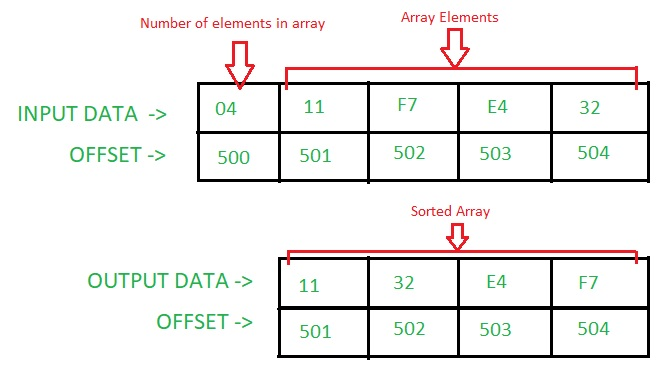# 8086 program for selection sort

• Last Updated : 22 May, 2018

Problem – Write an assembly language program in 8086 microprocessor to sort a given array of n numbers using Selection Sort.

Assumptions – The number of elements in the array is stored at offset 500. The array starts from offset 501.

Example –Algorithm –

1. We first find the smallest number in the array.
2. Swap the smallest number from the first element of the array.
3. Keep repeating the process till all elements are traversed.

Program –

OffsetMnemonicsComment
400MOV DI, 501DI < – 501
403MOV SI, 500SI < – 500
406MOV CL, [SI]CL < – [SI]
408XOR CH, CHCH < – CH ^(XOR) CH
40AINC SISI < – SI+0001
40BDEC CXCX < – CX-0001
40CMOV BX, SIBX < – SI
40EMOV AH, CLAH < – CL
410INC AHAH < – AH+01
412MOV AL, [SI]AL < – [SI]
414INC SISI < – SI+0001
415DEC AHAH < – AH-01
417CMP AL, [SI]AL-[SI]
419JC 41FIf Carry Flag = 1, goto offset 41F
41BMOV AL, [SI]AL < – [SI]
41DMOV BX, SIBX < – SI
41FINC SISI < – SI+0001
420DEC AHAH < – AH-01
422JNZ 417If Zero Flag = 0, goto offset 417
424MOV DL, [BX]DL < – [BX]
426XCHG DL, [DI]DL < – > [DI]
428XCHG DL, [BX]DL < – > [BX]
42AINC DIDI < – DI+0001
42BMOV SI, DISI < – DI
42DLOOP 40CCX < – CX-0001; If Zero Flag = 0, goto offset 40C.
42FHLTEnd of program.

Explanation – Registers AH, AL, BX, CX, DL, SI, DI are used for general purpose:

```AL - Stored the smallest number
AH - Stores the counter for the inner loop
BX - Stores the offset of the smallest
number of each iteration of the outer loop
CX - Stores the counter for the outer loop
DL - Helps in swapping the elements
SI - Pointer
DI - Pointer ```
1. MOV SI, 500: stores 0500 in SI.
2. MOV CL, [SI]: stores the content at offset SI in CL.
3. XOR CH, CH: stores the result of logical operation XOR b/w CH and CH in CH.
4. INC SI: increase the value of SI by 1.
5. DEC CX: decrease the value of CX by 1.
6. MOV AH, CL: stores the contents of CL in AH.
7. CMP AL, [SI]: compares the content of AL with content at offset SI. If AL < [SI] – Sets Carry Flag(i.e. Carry Flag = 1).
8. JC 41F: jumps to offset 041F, if carry flag is set(1).
9. JNZ 417: jumps to offset 0417, if zero flag is reset(0).
10. XCHG DL, [BX]: swaps the content of DL with content at offset BX.
11. LOOP 40C: decrease the value of CX by 1 and check whether Zero Flag is set(1) or not. If Zero Flag is reset(0), then it jumps to offset 040C.
12. HLT: terminates the program.
My Personal Notes arrow_drop_up